# ISEE Upper Level Quantitative : How to find circumference

## Example Questions

← Previous 1

### Example Question #1 : How To Find Circumference

A giant clock has a minute hand five yards in length. Since noon, the tip of the minute hand has traveled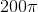feet. Which is the greater quantity?

(A) The amount of time that has passed since noon

(B) The amount of time until midnight

(A) and (B) are equal

It is impossible to determine which is greater from the information given

(B) is greater

(A) is greater

(A) is greater

Explanation:

Five yards is equal to fifteen feet, which is the length of the minute hand. Subsequently, fifteen feet is the radius of the circle traveled by its tip in one hour; the circumference of this circle istimes this, or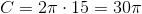feet.

In one six-hour period, the minute hand revolves six times, so its tip travles six times the circumference, or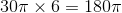The clock has traveled farther than this, so the time is later than 6:00 PM, and more time has elapsed since noon than is left until midnight. This makes (A) greater.

### Example Question #2 : How To Find Circumference

Compare the two quantities:

Quantity A: The area of a circle with radiusQuantity B: The circumference of a circle with radius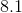The quantity in Column A is greater.

The relationship cannot be determined from the information given.

The two quantities are equal.

The quantity in Column B is greater.

The quantity in Column A is greater.

Explanation:

Recall for this question that the formulae for the area and circumference of a circle are, respectively: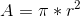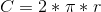For our two quantities, we have:

Quantity A: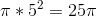Quantity B: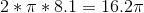Therefore, quantity A is greater.

### Example Question #3 : How To Find Circumference

The circumferences of eight circles form an arithmetic sequence. The smallest circle has radius two inches; the second smallest circle has radius five inches. Give the radius of the largest circle.

3 feet 10 inches

1 foot, 11 inches

2 feet

2 feet, 1 inch

4 feet 2 inches

1 foot, 11 inches

Explanation:

The circumference of a circle can be determined by multiplying its radius by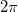, so the circumferences of the two smallest circles are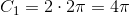and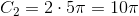The circumferences form an arithmetic sequence with common difference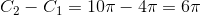The circumference of a circle can therefore be found using the formula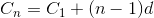where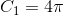and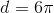; we are looking for that of the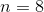th smallest circle, so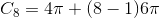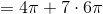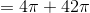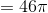Since the radius of a circle is the circumference of the circle divided by, the radius of this eighth circle is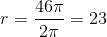inches, or 1 foot 11 inches.

### Example Question #4 : How To Find Circumference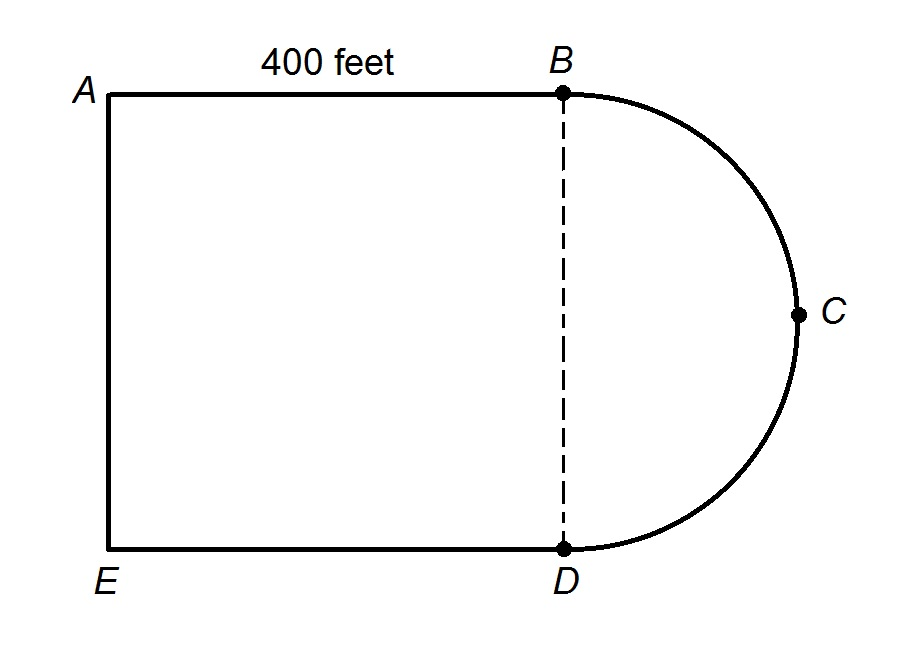The track at James Buchanan High School is shown above; it is comprised of a square and a semicircle.

Diane wants to run two miles. If she begins at Point A and begins running counterclockwise, when she is finished, which of the five points will she be closest to?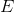Explanation:

First, it is necessary to know the length of the semicircle connecting Points B and D, which has diameter 400 feet; this length is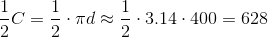feet.

The distance around the track is about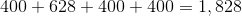feet.

Diane wants to run two miles, or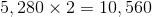feet.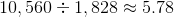circuits around the track.

Equivalently, she will run the track 5 complete times for a total of about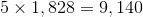feet,

so she will have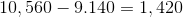feet to go.

She is running counterclockwise, so she will proceed from Point A to Point D, running another 800 feet, leaving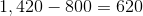feet.

She will almost, but not quite, finish the 628 feet from Point D to Point B.

The correct response is Point B.

### Example Question #161 : Geometry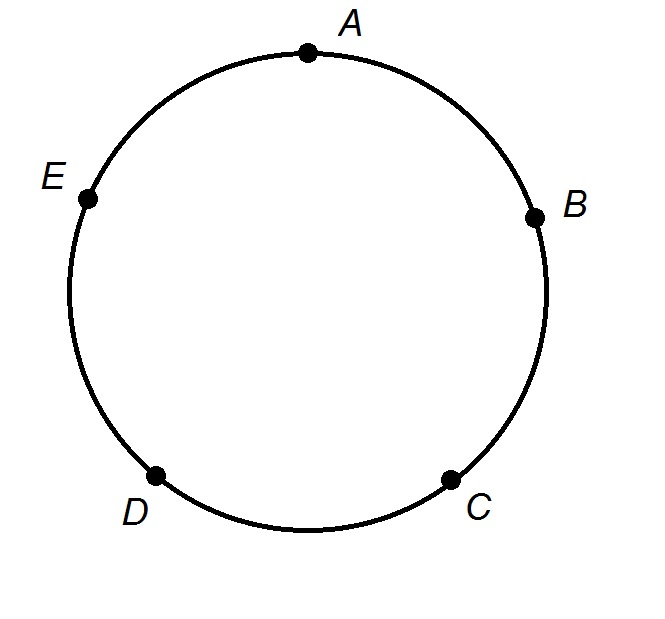The track at Monroe Elementary School is a perfect circle of radius 400 feet, and is shown in the above figure.

Evan and his younger brother Mike both start running from Point A. Evan runs counterclockwise, running once around the track and then on to Point E; Mike runs clockwise, meeting Evan at Point E and stopping.

Which of the following is the greater quantity?

(a) Twice Mike's average speed.

(b) Evan's average speed.

(Assume the five points are evenly spaced)

(b) is greater

It is impossible to tell which is greater from the information given

(a) and (b) are equal

(a) is greater

(b) is greater

Explanation:

It is not actually necessary to know the radius or length of the track if we know the points are equally spaced. Evan runs once around the track counterclockwise and then on to Point E, which is the next point after A; this means he runs around the track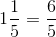times. Mike runs around the track clockwise from Point A to Point E, in the same time, meaning he runs around the tracktimes.

Therefore, Evan's speed is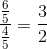times Mike's speed. As a result, Twice Mike's speed would be greater than Evan's speed, making (b) the greater.

### Example Question #6 : How To Find CircumferenceThe track at Monroe High School is a perfect circle of radius 600 feet, and is shown in the above figure.

Jerry begins his one-mile run at Point A, then runs counterclockwise around the track. At the end of his one-mile run, which is the greater quantity?

(a) The additional distance he would have to run if he were to continue to run counterclockwise to Point A.

(b) The additional distance he would have to run if he were to turn back and run clockwise to Point A.

It is impossible to tell from the information given

(b) is greater

(a) and (b) are equal

(a) is greater

(a) is greater

Explanation:

The circumference of a circle with radius 600 feet is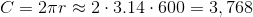feet.

A one mile run would be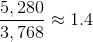times the length of the track.

Therefore, Jerry's run takes him around the track once, and about 0.4 times the length of the track. Since he is running counterclockwise, but has not made it halfway around the track yet, the longer of the two paths is to proceed counterclockwise and run the remaining 0.6 of the track. This makes (a) greater.

### Example Question #7 : How To Find CircumferenceThe track at Simon Bolivar High School is a perfect circle of radius 500 feet, and is shown in the above figure. Manuel starts at point C, runs around the track counterclockwise three times, and continues to run clockwise until he makes it to point D. Which of the following comes closest to the number of miles Manuel has run?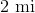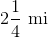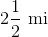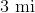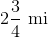Explanation:

The circumference of a circle with radius 500 feet is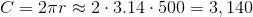feet.

Manuel runs this distance three times, then he runs from Point C to D, which is about four-fifths of this distance. Therefore, Manuel's run will be about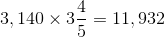feet.

Divide by 5,280 to convert to miles: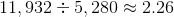,

making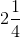miles the response closest to the actual running distance.

### Example Question #2 : How To Find Circumference

The radii of six circles form an arithmetic sequence. The radius of the second-smallest circle is twice that of the smallest circle. Which of the following, if either, is the greater quantity?

(a) The circumference of the largest circle

(b) Twice the circumference of the third-smallest circle

(a) is greater

It is impossible to tell from the information given

(b) is greater

(a) and (b) are equal

(a) and (b) are equal

Explanation:

Call the radius of the smallest circle. The radius of the second-smallest circle is then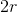, and the common difference of the radii is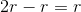.

The radii of the six circles are, from least to greatest: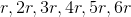The largest circle has circumference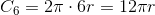The third-smallest circle has circumference: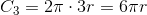Twice this is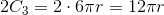The circumference of the sixth circle is equal to twice that of the third-smallest  circle, so the correct choice is that that (a) and (b) are equal.

### Example Question #9 : How To Find Circumference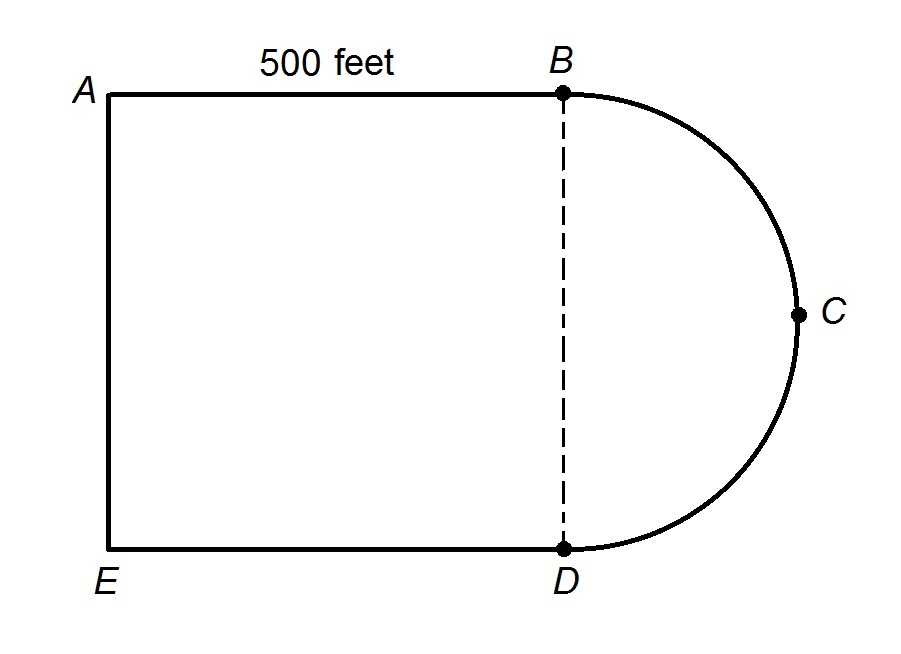The track at Truman High School is shown above; it is comprised of a square and a semicircle.

Veronica begins at Point A, runs three times around the track counterclockwise, and continues until she reaches Point B. Which of the following comes closest to the distance Veronica runs?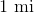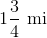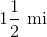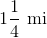Explanation:

First, it is necessary to know the length of the semicircle connecting Points B and D, which has diameter 500 feet; this length is about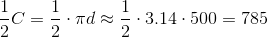feet.

The distance around the track is about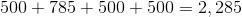feet.

Veronica runs around the track three complete times, for a distance of about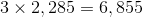feet.

She then runs from Point A to Point E, which is another 500 feet; Point E to Point D, which is yet another 500 feet, and, finally Point D to Point B, for a final 785 feet. The total distance Veronica runs is about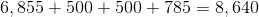feet.

Divide by 5,280 to convert to miles: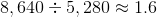The closest answer is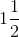miles.

### Example Question #161 : Plane GeometryThe track at Monroe High School is a perfect circle of radius 600 feet, and is shown in the above figure. Quinnella wants to run around the track for one and a half miles. If Quinnella starts at point C and runs counterclockwise, which of the following is closest to the point at which she will stop running?

(Assume the five points are evenly spaced)

Between Points E and A

Between Points A and B

Between Points D and E

Between Points C and D

Between Points B and C

Between Points B and C

Explanation:

A circle of radius 600 feet will have a circumference offeet.

Quinnella will run one and a half miles, or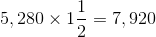feet,

which is about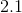times the circumference of the circle.

Quinnella will run around the track twice, returning to Point C; she will not quite make it to Point B a third time, since that is one-fifth of the track, or 0.2. The correct response is that she will be between Points B and C.

← Previous 1